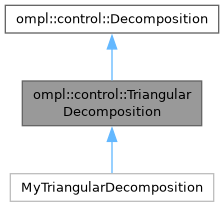ompl::control::TriangularDecomposition Class Reference

A TriangularDecomposition is a triangulation that ignores obstacles. More...

#include <ompl/extensions/triangle/TriangularDecomposition.h>

Inheritance diagram for ompl::control::TriangularDecomposition:[legend]

struct  Polygon

struct  Triangle

struct  Vertex

## Public Member Functions

TriangularDecomposition (const base::RealVectorBounds &bounds, std::vector< Polygon > holes=std::vector< Polygon >(), std::vector< Polygon > intRegs=std::vector< Polygon >())
Creates a TriangularDecomposition over the given bounds, which must be 2-dimensional. The underlying mesh will be a conforming Delaunay triangulation. The triangulation will ignore any obstacles, given as a list of polygons. The triangulation will respect the boundaries of any regions of interest, given as a list of polygons. No two obstacles may overlap, and no two regions of interest may overlap.

int getNumRegions () const override
Returns the number of regions in this Decomposition.

double getRegionVolume (int triID) override
Returns the volume of a given region in this Decomposition.

void getNeighbors (int triID, std::vector< int > &neighbors) const override
Stores a given region's neighbors into a given vector.

int locateRegion (const base::State *s) const override
Returns the index of the region containing a given State. Most often, this is obtained by first calling project(). Returns -1 if no region contains the State.

void sampleFromRegion (int triID, RNG &rng, std::vector< double > &coord) const override
Samples a projected coordinate from a given region.

void setup ()

int getNumHoles () const

int getNumRegionsOfInterest () const

const std::vector< Polygon > & getHoles () const

const std::vector< Polygon > & getAreasOfInterest () const

int getRegionOfInterestAt (int triID) const
Returns the region of interest that contains the given triangle ID. Returns -1 if the triangle ID is not within a region of interest.

void print (std::ostream &out) constPublic Member Functions inherited from ompl::control::Decomposition
Decomposition (int dim, const base::RealVectorBounds &b)
Constructor. Creates a Decomposition with a given dimension and a given set of bounds. Accepts as an optional argument a given number of regions.

virtual int getDimension () const
Returns the dimension of this Decomposition.

virtual const base::RealVectorBoundsgetBounds () const
Returns the bounds of this Decomposition.

virtual void project (const base::State *s, std::vector< double > &coord) const =0
Project a given State to a set of coordinates in R^k, where k is the dimension of this Decomposition.

virtual void sampleFullState (const base::StateSamplerPtr &sampler, const std::vector< double > &coord, base::State *s) const =0
Samples a State using a projected coordinate and a StateSampler.

## Protected Member Functions

virtual int createTriangles ()
Helper method to triangulate the space and return the number of triangles.

## Protected Attributes

std::vector< Triangletriangles_

std::vector< Polygonholes_

std::vector< PolygonintRegs_

std::vector< int > intRegInfo_
Maps from triangle ID to index of Polygon in intReg_ that contains the triangle ID. Maps to -1 if the triangle ID is not in a region of interest.

double triAreaPct_Protected Attributes inherited from ompl::control::Decomposition
int dimension_

base::RealVectorBounds bounds_

## Detailed Description

A TriangularDecomposition is a triangulation that ignores obstacles.

Definition at line 55 of file TriangularDecomposition.h.

The documentation for this class was generated from the following files: